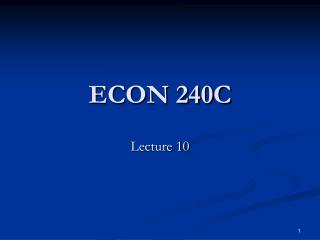# ECON 240C - PowerPoint PPT PresentationDownload PresentationECON 240C

ECON 240CDownload Presentation## ECON 240C

- - - - - - - - - - - - - - - - - - - - - - - - - - - E N D - - - - - - - - - - - - - - - - - - - - - - - - - - -
##### Presentation Transcript

1. ECON 240C Lecture 10

2. Outline • Box-Jenkins Passengers • Displaying the Forecast • Recoloring • ARTWO’s and cycles • Time series • Autocorrelation function • Private housing Starts- Single Units • Review: Unit Roots • Midterm 2002

3. Forecasting Seasonal Difference in the Fractional Change • Estimation period: 1949.01 – 1960.12 • Forecast period: 1961.01 – 1961.12

4. Eviews forecast command window

5. Eviews plot of forecast plus or minus two standard errors Of the forecast

6. Eviews spreadsheet view of the forecast and the standard Error of the forecast

7. Using the Quick Menu and the show command to create Your own plot or display of the forecast

8. Note: EViews sets the forecast variable equal to the observed Value for 1949.01-1960.12.

9. To Differentiate the Forecast from the observed variable …. • In the spread sheet window, click on edit, and copy the forecast values for 1961.01-1961.12 to a new column and paste. Label this column forecast.

10. Note: EViews sets the forecast variable equal to the observed Value for 1949.01-1960.12.

11. Displaying the Forecast • Now you are ready to use the Quick menu and the show command to make a more pleasing display of the data, the forecast, and its approximate 95% confidence interval.

12. Qick menu, show command window

13. Recoloring • The seasonal difference of the fractional change in airline passengers may be appropriately pre-whitened for Box-Jenkins modeling, but it is hardly a cognitive or intuitive mode for understanding the data. Fortunately, the transformation process is reversible and we recolor, i.e put back the structure we removed with the transformations by using the definitions of the transformations themselves

14. Recoloring • Summation or integration is the opposite of differencing. • The definition of the first difference is: (1-Z) x(t) = x(t) –x(t-1) • But if we know x(t-1) at time t-1, and we have a forecast for (1-Z) x(t), then we can rearrange the differencing equation and do summation to calculate x(t): x(t) = x0(t-1) + Et-1 (1-Z) x(t) • This process can be executed on Eviews by using the Generate command

15. Recoloring • In the case of airline passengers, it is easier to undo the first difference first and then undo the seasonal difference. For this purpose, it is easier to take the transformations in the order, natural log, seasonal difference, first difference • Note: (1-Z)(1-Z12)lnBJPASS(t) = (1-Z12)(1-Z) lnBJPASS(t), i.e the ordering of differencing does not matter

16. Correlogram of Seasonal Difference in log of passengers. Note there is still structure, decay in the ACF, requiring A first difference to further prewhiten

17. As advertised, either order of differencing results in the Same pre-whitened variable

18. Using Eviews to Recolor • DSDlnBJP(t) = SDlnBJPASS(t) – SDBJPASS(t-1) • DSDlnBJP(1961.01) = SDlnBJPASS(1961.01) – SDlnBJPASS(1960.12) • So we can rearrange to calculate forecast values of SDlnBJPASS from the forecasts for DSDlnBJP • SDlnBJPASSF(1961.01) = DSDlnBJPF(1961.01) + SDlnBJPASS(1960.12) • We can use this formula in iterative fashion as SDlnBJPASSF(1961.01) = DSDlnBJPF(1961.01) + SDlnBJPASSF(1960.12), but we need an initial value for SDlnBJPASSF(1960.12) since this is the last time period before forecasting.

19. The initial value • This problem is easily solved by generating SDlnBJPASSF(1960.12) = SDlnBJPASS(1960.12)

20. Recoloring: Generating the forecast of the seasonal difference in lnBJPASS

21. Forecast of sdlnbjpa

22. Recoloring to Undo the Seasonal Difference in the Log of Passengers • Use the definition: SDlnBJPASS(t) = lnBJPASS(t) – lnBJPASS(t-12), • Rearranging and putting in terms of the forecasts lnBJPASSF(1961.01) = lnBJPASS(1960.12) + SDlnBJPASSF(1961.01) • In this case we do not need to worry about initial values in the iteration because we are going back twelve months and adding the forecast for the seasonal difference

23. lnbjpassf

24. The Harder Part is Over • Once the difference and the seasonal difference have been undone by summation, the rest requires less attention to detail, plus double checking, to make sure your commands to Eviews were correct.

25. The Last Step • To convert the forecast of lnBJPASS to the forecast of BJPASS use the inverse of the logarithmic transformation, namely the exponential

26. Confidence Intervals • The confidence interval can be generated as: • Lnupper = lnbjpass + 2*sef • Lnlower = lnbjpassf-2*sef • And then exponentiated: • Upper= exp(lnupper) • Lower=exp(lnlower)

27. Part I: ARTWO’s and Cycles • ARTWO(t) = b1 ARTWO(t-1) + b2 ARTWO(t-2) + WN(t) • ARTWO(t) = b1 ARTWO(t-1) + b2 ARTWO(t-2) is the homogenous deterministic part of the equation after dropping the stochastic part, WN(t). • Substitute y2-u for ARTWO(t-u) to obtain: • y2 =b1 y1 + b2 y0 • Or y2 - b1 y1 - b2 = 0, which is a quadratic

28. Note that the corresponding equation for the autocorrelation function has the same behavior: • r(2) = b1 r(1) + b2 r(0) • Let y2-u = r(2-u), then • y2 =b1 y1 + b2 y0 • The same homogeneous equation for the autocorrelation function as for the process, so if the process cycles so will the autocorrelation function

29. Simulated ARTWO • ARTWO = 0.6*ARTWO(-1) - 0.8*ARTWO(-2)+ WN

30. Estimated Coefficients: Simulated ARTWO

31. Privately Owned Housing Starts